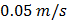# A uniform chain of lengthand massis lying on a smooth table and one third of its length is hanging vertically down over the edge of the table. Ifis acceleration due to gravity, the work required to pull the hanging part on to the table is a)b)c)d)## Question ID - 51072 :- A uniform chain of lengthand massis lying on a smooth table and one third of its length is hanging vertically down over the edge of the table. Ifis acceleration due to gravity, the work required to pull the hanging part on to the table is a)b)c)d)3537

(d)[Given ]

Next Question :
 Four smooth steel balls of equal mass at rest are free to move along a straight line without friction. The first ball is given a velocity of. It collides head on with the second elastically, the second one similarly with the third and so on. The velocity of the last ball is a)b)c)d)